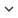ENGArticle
Article 440
Author/s
• G. Espiñeira, D. Nagy, G. Indalecio, A. J. García-Loureiro, K. Kalna and N. Seoane
DOI
Source
• IEEE Electron Device Letters, 2019 - Q1

# Impact of Gate Edge Roughness Variability on FinFET and Gate-All-Around Nanowire FET

The effect of gate edge roughness (GER) in the sub-threshold region is studied for two state-of-the-art architectures: a 10.7-nm Si FinFET and a 10-nm Si gate-all-around (GAA) nanowire (NW) FET using an in-house 3D quantum-corrected drift-diffusion simulation tool. The GER is applied to the device gate using the characteristic values of root-mean-square amplitude and correlation length (CL). The GER-induced variability results in a standard deviation (sigma) for the threshold voltage (Vt ) of 7 mV for the FinFET when CL/Gate Perimeter = 0.66 and RMS = 0.80 nm, which is 20% greater than that of the GAA NW FET. GER is a less damaging source of variability than metal grain granularity (MGG), line edge roughness (LER), and random dopants (RD) for both devices. When compared to LER variations, sigma(Vt) due to the GER is 62% and 86% lower for the FinFET and GAA NW FET, respectively. However, although GER affects the FinFET more than the GAA NW FET, the combined variability effect of GER, MGG, LER, and RD ( sigma(Vt,comb) ) on the FinFET is 30 mV, a value approximately 50% smaller than that of the GAA NW FET.
Keywords: FinFET, gate-all-around nanowire FET, gate edge roughness, variability